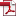• KSII Transactions on Internet and Information Systems
Monthly Online Journal (eISSN: 1976-7277)

# A hybrid model of regional path loss of wireless signals through the wall

Vol. 16, No. 9, September 30, 202210.3837/tiis.2022.09.020, Download Paper (Free):#### Abstract

Wall obstruction is the main factor leading to the non-line of sight (NLoS) error of indoor localization based on received signal strength indicator (RSSI). Modeling and correcting the path loss of the signals through the wall will improve the accuracy of RSSI localization. Based on electromagnetic wave propagation theory, the reflection and transmission process of wireless signals propagation through the wall is analyzed. The path loss of signals through wall is deduced based on power loss and RSSI definition, and the theoretical model of path loss of signals through wall is proposed. In view of electromagnetic characteristic parameters of the theoretical model usually cannot be accurately obtained, the statistical model of NLoS error caused by the signals through the wall is presented based on the log-distance path loss model to solve the parameters. Combining the statistical model and theoretical model, a hybrid model of path loss of signals through wall is proposed. Based on the empirical values of electromagnetic characteristic parameters of the concrete wall, the effect of each electromagnetic characteristic parameters on path loss is analyzed, and the theoretical model of regional path loss of signals through the wall is established. The statistical model and hybrid model of regional path loss of signals through wall are established by RSSI observation experiments, respectively. The hybrid model can solve the problem of path loss when the material of wall is unknown. The results show that the hybrid model can better express the actual trend of the regional path loss and maintain the pass loss continuity of adjacent areas. The validity of the hybrid model is verified by inverse computation of the RSSI of the extended region, and the calculated RSSI is basically consistent with the measured RSSI. The hybrid model can be used to forecast regional path loss of signals through the wall.